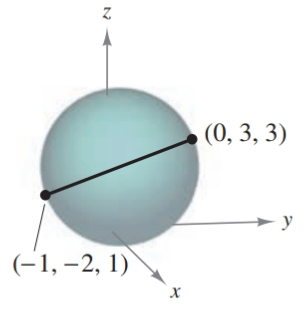Chapter 7.1, Problem 32E### Calculus: An Applied Approach (Min...

10th Edition
Ron Larson
ISBN: 9781305860919

#### Solutions

Chapter
Section### Calculus: An Applied Approach (Min...

10th Edition
Ron Larson
ISBN: 9781305860919
Textbook Problem
1 views

# Finding the equation of a Sphere In Exercises 29–38, find the standard equation of the sphere with the given characteristics. See Examples 4 and 5.To determine

To calculate: The standard equation of sphere from the provided figure,Explanation

Given Information:

The provided figure,

Formula used:

The standard equation of a sphere with centre at (h,k,j) and radius r is,

(xh)2+(yk)2+(zj)2=r2

Calculation:

From the provided figure, the endpoints of the diameter of the sphere are (0,3,3) and (1,2,1).

Since, the midpoint of the diameter of a sphere is the centre of sphere. So, by the use of midpoint formula to find the centre of sphere,

Midpoint=(x1+x22,y1+y22,z1+z22)=(0+(1)2,3+(2)2,3+12)=(12,12,2)

Since, the distance between the centre and circumference point of the sphere is the radius of the sphere. So, to find the radius of the sphere by the use of distance formula,

r=(x2x1)2+(y2y1)2+(z2z1)2=

### Still sussing out bartleby?

Check out a sample textbook solution.

See a sample solution

#### The Solution to Your Study Problems

Bartleby provides explanations to thousands of textbook problems written by our experts, many with advanced degrees!

Get Started

#### Find more solutions based on key concepts